# Bayesian Hyperparameter Optimization with MLflow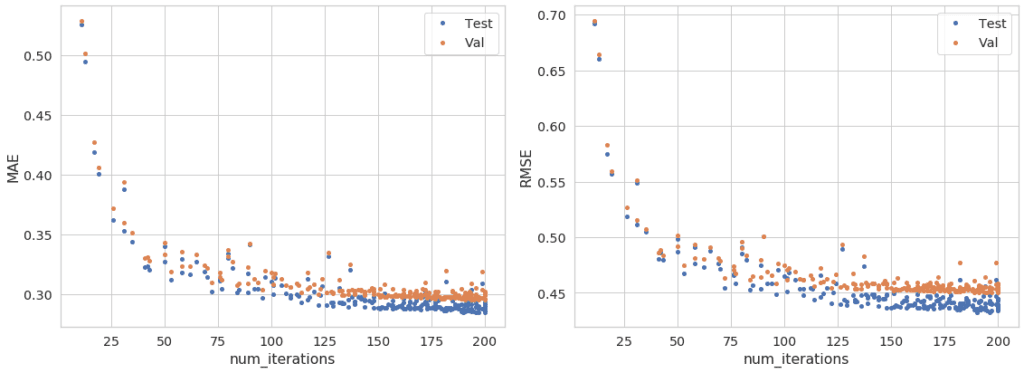Bayesian hyperparameter optimization is a bread-and-butter task for data scientists and machine-learning engineers; basically, every model-development project requires it.  Hyperparameters are the parameters (variables) of machine-learning models that are not learned from data, but instead set explicitly prior to training – think of them as knobs that need to be fiddled with in order to find the best model for a given task. Ultimately, regardless of what you’re doing with machine learning, hyperparameters should be optimized.

Traditional hyperparameter optimization used a grid search or random search to sample various combinations of hyperparameters and empirically evaluate model performance. By trying out many combinations of hyperparameters, experimenters can usually get a good sense of where to set parameters to achieve optimal performance. Recent research has yielded new algorithms that intelligently narrow the search space as more and more combinations are tested. In other words, once we’ve run some experiments on different hyperparameter combinations and estimated model performance, we start to get a sense of ranges for each parameter where we should focus future experiments. Versions of these narrowing techniques include Bayesian Optimization, Tree of Parazen Estimators (TPE), and Bandit Algorithms. In this blog post, we use a Python library called Hyperopt to direct our hyperparameter search, in particular, because its Spark integration makes parallelization of experiments straightforward.

One particular challenge in hyperparameter optimization is tracking the sheer number of experiments. As we refine our experiments and run new searches, the bookkeeping of all these results can become maddening. Enter MLflow. MLflow serves a handful of important purposes in machine-learning projects – environment management, streamlining of deployments, artifact persistence – but in the context of hyperparameter optimization, it is particularly useful for experiment tracking. Using MLflow, an experimenter can log one or several metrics and parameters with just a single API call. Further, MLflow has logging plugins for the most common machine-learning frameworks (Keras, TensorFlow, XGBoost, LightGBM, etc.) to automate the persistence of model artifacts for future deployment. And when experimenting in a Databricks environment, MLflow’s tracking servers and storage are configured automatically with every notebook, making it trivially easy to take advantage of this functionality.

While experiment tracking is useful in the context of Bayesian hyperparameter optimization, it is more generally an essential component of machine-learning operations (MLOps).  A good MLOps pipeline enables reproducible research by keeping track of experiments automatically so that data scientists can focus on innovation.  This MLflow tutorial shows how data scientists can diligently log their experiments with minimal overhead.

## MLflow tutorial: Tracking experiments

When working in Databricks, a simple user interface allows us to configure a cluster to gain access to the rich parallelization API of Apache Spark. All Databricks notebooks have tight integration with MLflow without any further configuration. On an otherwise default cluster configuration, we’re using Databricks Runtime 7 ML to define our Python environment, which happens to include all of the libraries necessary for this demo.

In this MLflow tutorial, our Databricks notebook opens up by downloading the dataset used for demonstration purposes. There’s nothing too exciting about the dataset; we’re focusing on the techniques here, not the novelty of the use case. To keep it simple, we’re using the California Housing Dataset accessible through Scikit-learn API. In short, the dataset includes roughly 20,000 examples of California regions with area median home price as a regression target and nine features for model input. The code to fetch the dataset; extract the feature matrix (x) and target vector (y); and define a train/test split is as follows:

```import pandas as pd
from sklearn import datasets
from sklearn import model_selection

data = datasets.fetch_california_housing()
x = pd.DataFrame(data['data'], columns=data['feature_names'])
y = pd.Series(data['target'])

x_train, x_test, y_train, y_test = model_selection.train_test_split(
x, y, test_size=0.2, random_state=42)```

Once we have our data prepared, we want to define the metrics that we will use to track model performance for our experiments. It is helpful to wrap those up into a single function that returns a collection of metrics based on ground truth (actual) and model predictions (pred) for the target variable, like so:

```from typing import Dict

import numpy as np
from sklearn import metrics

def regression_metrics(actual: pd.Series,
pred: pd.Series) -> Dict:
"""Return a collection of regression metrics as a Series.

Args:
actual: series of actual/true values
pred: series of predicted values

Returns:
Series with the following values in a labeled index:
MAE, RMSE
"""
return {
"MAE": metrics.mean_absolute_error(actual, pred),
"RMSE": np.sqrt(metrics.mean_squared_error(actual, pred))}```

The returned metrics are Mean Absolute Error (MAE) and Root Mean Square Error (RMSE).

Now we start to get to the meat of our ML training task by defining a function that can fit a machine-learning model. In this case, we’re training a Gradient Boosted Model (GBM) with LightGBM. If you’re familiar with XGBoost, this approach is nearly identical. But MLflow has logging plugins for TensorFlow and Keras and many other modeling frameworks, so there are many options here. Even if a plugin is not built into MLflow for a more exotic model type, it is straightforward to log parameters, metrics, and artifacts manually. In our case, we first use cross validation to determine the metric scores for our training set. These are the actual metric values we will optimize. The function below takes advantage of the Scikit-learn interface of LightGBM and the convenience of sklearn.model_selection.cross_val_predict() to generate predictions for the entire training set using five-fold cross validation; that is, we fit five different models on five distinct training samples with statistically disjoint validation samples.  However, we do not log the parameters of these specific models. Once validation scores are measured for a given set of hyperparameters, we enable automatic logging with MLflow and refit a model with the same hyperparameters on the entire training set. For comparison, we determine and log metrics for the test set as well, though a data scientist should never optimize the model based on scores from the test set. The test metrics are used for downstream analysis to ensure that our model has not overfit for our training set. More on that later, but without further ado, here is our function for model fitting experiments and tracking outcomes:

```import mlflow
import mlflow.lightgbm
from sklearn import model_selection
from typing import Any
from typing import Dict
from typing import Union
from typing import Tuple
import lightgbm

def fit_and_log_cv(x_train: Union[pd.DataFrame, np.array],
y_train: Union[pd.Series, np.array],
x_test: Union[pd.DataFrame, np.array],
y_test: Union[pd.Series, np.array],
params: Dict[str, Any],
nested: bool = False) -> Tuple[Dict[str, Any], Dict[str, Any]]:
"""Fit a model and log it along with train/CV metrics.

Args:
x_train: feature matrix for training/CV data
y_train: label array for training/CV data
x_test: feature matrix for test data
y_test: label array for test data
nested: if true, mlflow run will be started as child
of existing parent
"""
with mlflow.start_run(nested=nested) as run:
# Fit CV models; extract predictions and metrics
print(type(params))
print(params)
model_cv = lightgbm.LGBMRegressor(**params)
y_pred_cv = model_selection.cross_val_predict(model_cv, x_train, y_train)
metrics_cv = {
f"val_{metric}": value
for metric, value in regression_metrics(y_train, y_pred_cv).items()}

# Fit and log full training sample model; extract predictions and metrics
mlflow.lightgbm.autolog()
dataset = lightgbm.Dataset(x_train, label=y_train)
model = lightgbm.train(params=params, train_set=dataset)
y_pred_test = model.predict(x_test)
metrics_test = {
f"test_{metric}": value
for metric, value in regression_metrics(y_test, y_pred_test).items()}

metrics = {**metrics_test, **metrics_cv}
mlflow.log_metrics(metrics)
return metrics```

Logging metrics to MLflow means that we can check out the results of our experiments using the MLflow UI, accessible from the top right corner of our notebook interface: Thus we have a cleanly defined training experiment function that takes train/test datasets and a set of hyperparameters, and outputs a set of metrics that can be used for evaluation or analysis. Note also the nested parameter to mlflow.start_run() that allows an MLflow run to be a nested child of another run, so that a hierarchy of runs can be linked for downstream analysis.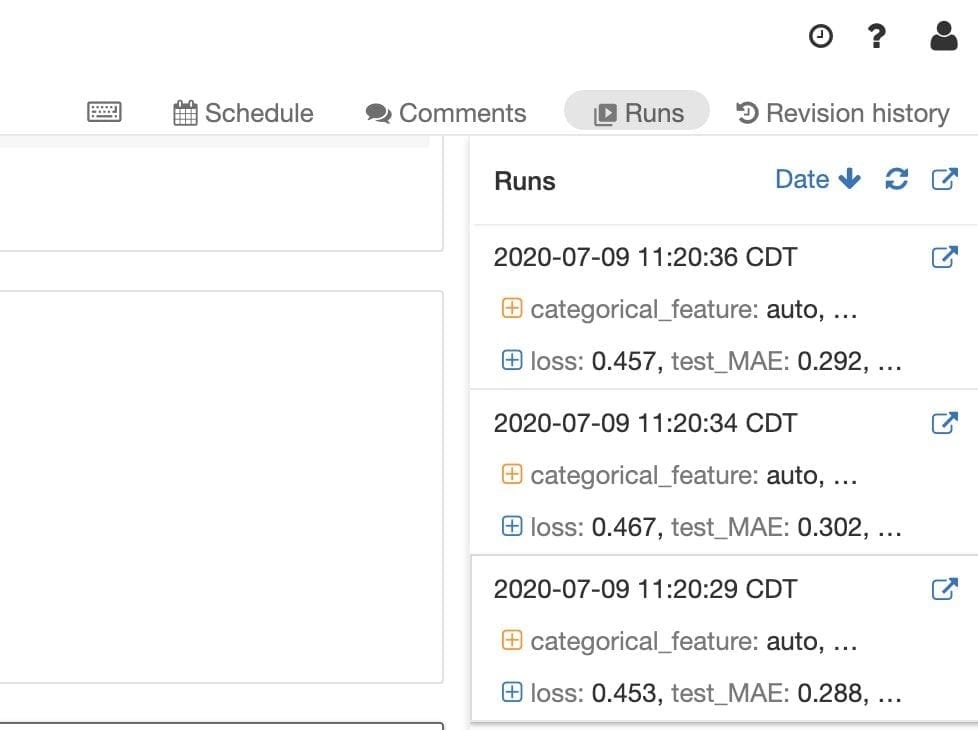For any given run tracked in MLflow, we can see the logged metrics and parameters. The parameters and loss metric were logged automatically by mlflow.lightgbm.autolog().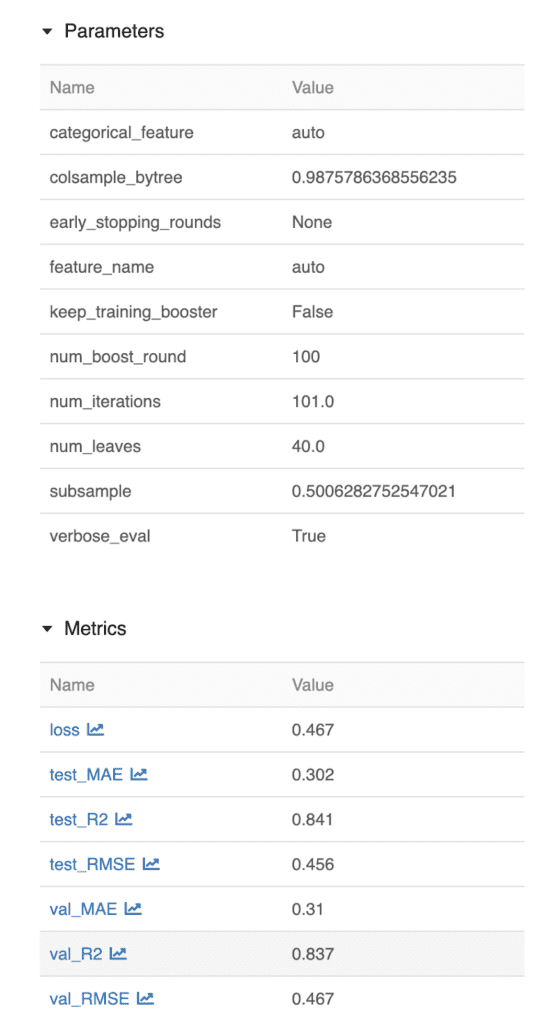Perhaps most conveniently, MLflow’s automatic logging also captures artifacts from our model training. In the MLflow interface, we can see that it has stored a serialized copy of the model trained for this experiment, as well as feature-importance data for potential analysis.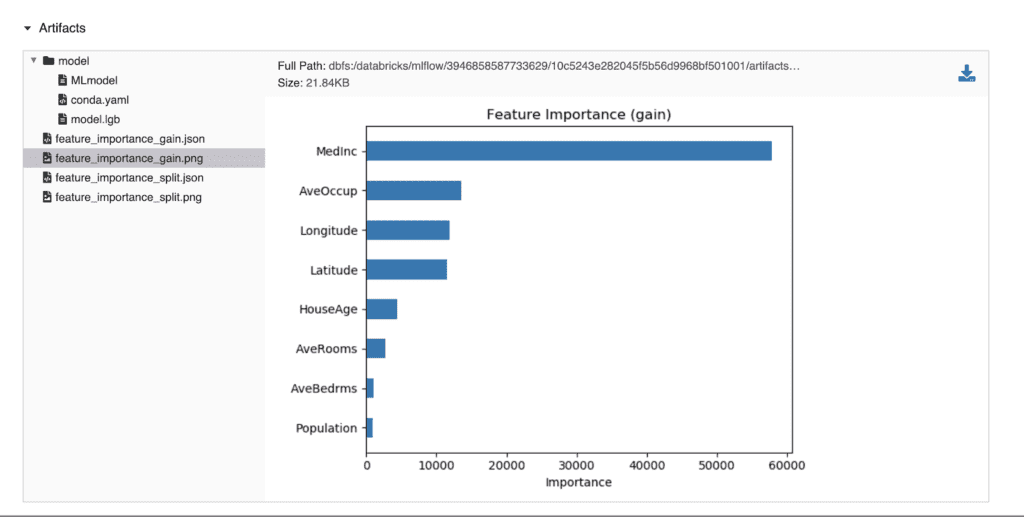## Bayesian Hyperparameter Optimization with Hyperopt

With a great experiment tracking and logging setup in hand, we can move on to optimizing hyperparameters. The beauty of Hyperopt is that it doesn’t care what sort of function you’re optimizing. All we need to do is create a function reference that takes parameters as input, and returns the optimization metric to narrow the search for subsequent sampling of parameters. It is helpful, however, to prepare the optimization function to be returned by a higher-level outer function. The outer function is used to close over some common variables (train/test data and metric choice) that are the same for every hyperparameter sample/experiment, leaving the inner function to simply take the parameters as input and return the metric to Hyperopt as output.

```import hyperopt

def build_train_objective(x_train: Union[pd.DataFrame, np.array],
y_train: Union[pd.Series, np.array],
x_test: Union[pd.DataFrame, np.array],
y_test: Union[pd.Series, np.array],
metric: str):
"""Build optimization objective function fits and evaluates model.

Args:
x_train: feature matrix for training/CV data
y_train: label array for training/CV data
x_test: feature matrix for test data
y_test: label array for test data
metric: name of metric to be optimized

Returns:
Optimization function set up to take parameter dict from Hyperopt.
"""

def train_func(params):
"""Train a model and return loss metric."""
metrics = fit_and_log_cv(
x_train, y_train, x_test, y_test, params, nested=True)
return {'status': hyperopt.STATUS_OK, 'loss': metrics[metric]}

return train_func```

The last thing to consider before starting to run hundreds of hyperparameter combination experiments is how to record the combination with the optimal results. Here, we define a function that searches over the previously evaluated experiments to find the one with the best metric and log the results to MLflow. These results are logged to the parent MLflow run, under which all of the individual experiments are nested as child runs. Here is a handy function to serve that purpose:

```def log_best(run: mlflow.entities.Run,
metric: str) -> None:
"""Log the best parameters from optimization to the parent experiment.

Args:
run: current run to log metrics
metric: name of metric to select best and log
"""

client = mlflow.tracking.MlflowClient()
runs = client.search_runs(
[run.info.experiment_id],
"tags.mlflow.parentRunId = '{run_id}' ".format(run_id=run.info.run_id))

best_run = min(runs, key=lambda run: run.data.metrics[metric])

mlflow.set_tag("best_run", best_run.info.run_id)
mlflow.log_metric(f"best_{metric}", best_run.data.metrics[metric])```

Now we can put it all together and run our hyperparameter search experiments. Note that aside from our train/test data, we haven’t even defined any global variables in our notebook. This is where we start to do that to configure our search. We specify 200 iterations; meaning that we will experiment with 200 different combinations of hyperparameters. The metric of choice is selected as RMSE on the validation sample. And finally, we specify a parallelism of 8, meaning that we will run 8 experiments simultaneously. There’s not a lot of magic to selecting the number of iterations (experiments) and parallelism, but keep in mind that as parallelism increases, these narrowing search algorithms can lose some ability to refine the space for subsequent experiments. Quite simply, the more we do all at once, the less we can take advantage of what we’re learning as we go. The experiments are parallelized by Spark using Hyperopt, without any complicated configuration thanks to Databricks. We also define the search space as ranges of variables and how to sample them and configure our training objective with the train/test samples defined above.

```from hyperopt.pyll.base import scope

MAX_EVALS = 200
METRIC = "val_RMSE"
# Number of experiments to run at once
PARALLELISM = 8

space = {
'colsample_bytree': hyperopt.hp.uniform('colsample_bytree', 0.5, 1.0),
'subsample': hyperopt.hp.uniform('subsample', 0.05, 1.0),
# The parameters below are cast to int using the scope.int() wrapper
'num_iterations': scope.int(
hyperopt.hp.quniform('num_iterations', 10, 200, 1)),
'num_leaves': scope.int(hyperopt.hp.quniform('num_leaves', 20, 50, 1))
}

trials = hyperopt.SparkTrials(parallelism=PARALLELISM)
train_objective = build_train_objective(
x_train, y_train, x_test, y_test, METRIC)

with mlflow.start_run() as run:
hyperopt.fmin(fn=train_objective,
space=space,
algo=hyperopt.tpe.suggest,
max_evals=MAX_EVALS,
trials=trials)
log_best(run, METRIC)
search_run_id = run.info.run_id
experiment_id = run.info.experiment_id```

Here, we start the parent run, under which all of our individual experiments will be nested. Calling hyperopt.fmin() triggers the running of experiments and hyperparameter sampling. We then log the results of the best experiments and capture the run and experiment identifiers for downstream analysis.

## Analysis of Results

Now that we’ve run all of our experiments, we can start to take a peek at the results. Using the MLflow tracking API, it is easy to download metrics and parameters and populate a Pandas DataFrame for analytics.

```client = mlflow.tracking.MlflowClient()
runs = client.search_runs([experiment_id],
f"tags.mlflow.parentRunId = '{search_run_id}' ")
# Extract metrics and parameters
df_metrics = pd.DataFrame.from_records(
[{"run_id": run.info.run_id, **run.data.metrics, **run.data.params} for run in runs])```

Now we can start to dig into the results. First, we want to check that our various metrics aren’t competing with each other and that our train and test samples are showing the same trends. A quick way to do this is by using a Scatterplot Matrix from Seaborn. We define the metrics we want to look at, and make the plots using Seaborn’s API:

```import seaborn

eval_metrics = ["val_MAE", "val_RMSE", "test_MAE", "test_RMSE"]
seaborn.pairplot(df_metrics[eval_metrics])```

The code above yields the following figure: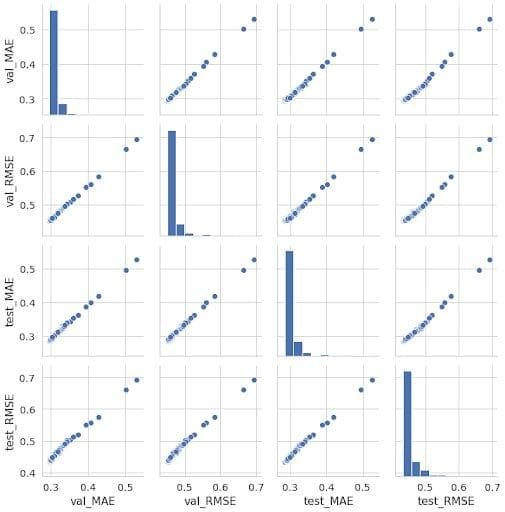The histograms on the diagonal show the one-dimensional distribution of each metric, but more importantly, we can see good correlation between the metrics. The MAE and RMSE metrics are correlated with each other, meaning that the models that give the best MAE will generally give the best RMSE; lower is better for both. But most importantly, we see good correlation between our test and validation metrics. This indicates that we are not seeing strong overfitting on our models – the models that give the best results on cross validation also give the best results on the test set.

To wrap up our basic analysis, we can also take a look at how the metrics are correlated with our parameters to understand which hyperparameters are actually contributing significantly to model performance. The code below loops over the parameters and metrics to generate some scatter plots using Matplotlib.

```from matplotlib import pyplot as plt

params = space.keys()
metric_names = ["MAE", "RMSE"]

for param in params:
fig = plt.figure(figsize=(16, 6))
for pane, metric in enumerate(metric_names):
plt.subplot(1, len(metric_names), pane + 1)
plt.plot(
df_metrics[param].astype(float), df_metrics[f"test_{metric}"],
'.', label="Test")
plt.plot(
df_metrics[param].astype(float), df_metrics[f"val_{metric}"],
'.', label="Val")
plt.xlabel(param)
plt.ylabel(metric)
plt.legend()
plt.tight_layout()```

Most of the plots generated are uninteresting because it turns out that our model performance has little dependence on all but one of the hyperparameters: num_iterations. Note that this is not the number of experiments, but instead the number of boosting rounds applied by LightGBM during training. Here’s the plot of interest:We can see here that increasing the number of boosting iterations generally improves the model performance by lowering the MAE and RMSE metrics, but only up to a point. Once the number of iterations increases beyond 150, the improvement largely flattens out. We can also see in this figure that our validation scores are generally a bit larger (worse) than the test scores. This is likely due to the fact that the training sample is effectively 25% larger than the five-fold cross-validation samples – more data generally makes a better model.

## Conclusion: MLOps doesn’t have to be difficult

The gold standard in MLOps is to enable data scientists to innovate while also ensuring that their work is ready for deployment.  We’ve seen here that MLflow can greatly simplify our efforts by tracking experiments, especially as we do hyperparameter optimization and the number of experiments grows into the hundreds or even thousands. MLflow also makes it easy to use track metrics, parameters, and artifacts when we use the most common libraries, such as LightGBM. Hyperopt has proven to be a good choice for sampling our hyperparameter space in an intelligent way, and makes it easy to parallelize with its Spark integration. All of these things come together seamlessly in Databricks, where Spark clusters are configured easily and MLflow is coupled automatically with every notebook.

Of course, the challenging part of data science is always adapting straightforward examples like this one to more complicated datasets and use cases. If you’d like a hand tackling your next problem, reach out to the Machine Learning Engineers and Data Scientists at phData. We’re here to help!Build a data-driven future with end-to-end services to architect, deploy, and support machine learning and data analytics.

## Snowflake